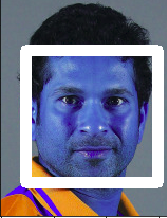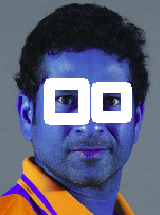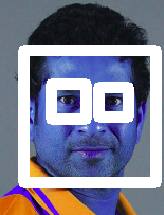Related Articles
Python | Haar Cascades for Object Detection
• Difficulty Level : Medium
• Last Updated : 25 Nov, 2019

Object Detection is a computer technology related to computer vision, image processing and deep learning that deals with detecting instances of objects in images and videos. We will do object detection in this article using something known as haar cascades.

Haar Cascade classifiers are an effective way for object detection. This method was proposed by Paul Viola and Michael Jones in their paper Rapid Object Detection using a Boosted Cascade of Simple Features .Haar Cascade is a machine learning-based approach where a lot of positive and negative images are used to train the classifier.

• Positive images – These images contain the images which we want our classifier to identify.
• Negative Images – Images of everything else, which do not contain the object we want to detect.

Requirements

• Make sure you have python, Matplotlib and OpenCV installed on your pc (all the latest versions).

Implementation

 `# Importing all required packages ` `import` `cv2 ` `import` `numpy as np ` `import` `matplotlib.pyplot as plt ``%` `matplotlib inline ` ` `  ` `  `# Read in the cascade classifiers for face and eyes ` `face_cascade ``=` `cv2.CascadeClassifier(``'../DATA / haarcascades / haarcascade_frontalface_default.xml'``) ` `eye_cascade ``=` `cv2.CascadeClassifier(``'../DATA / haarcascades / haarcascade_eye.xml'``) ` ` `  ` `  ` `  `# create a function to detect face ` `def` `adjusted_detect_face(img): ` `     `  `    ``face_img ``=` `img.copy() ` `     `  `    ``face_rect ``=` `face_cascade.detectMultiScale(face_img,  ` `                                              ``scaleFactor ``=` `1.2``,  ` `                                              ``minNeighbors ``=` `5``) ` `     `  `    ``for` `(x, y, w, h) ``in` `face_rect: ` `        ``cv2.rectangle(face_img, (x, y),  ` `                      ``(x ``+` `w, y ``+` `h), (``255``, ``255``, ``255``), ``10``)\ ` `         `  `    ``return` `face_img ` ` `  ` `  `# create a function to detect eyes ` `def` `detect_eyes(img): ` `     `  `    ``eye_img ``=` `img.copy()     ` `    ``eye_rect ``=` `eye_cascade.detectMultiScale(eye_img,  ` `                                            ``scaleFactor ``=` `1.2``,  ` `                                            ``minNeighbors ``=` `5``)     ` `    ``for` `(x, y, w, h) ``in` `eye_rect: ` `        ``cv2.rectangle(eye_img, (x, y),  ` `                      ``(x ``+` `w, y ``+` `h), (``255``, ``255``, ``255``), ``10``)         ` `    ``return` `eye_img ` ` `  `# Reading in the image and creating copies ` `img ``=` `cv2.imread(``'../sachin.jpg'``) ` `img_copy1 ``=` `img.copy() ` `img_copy2 ``=` `img.copy() ` `img_copy3 ``=` `img.copy() ` ` `  `# Detecting the face  ` `face ``=` `adjusted_detect_face(img_copy) ` `plt.imshow(face) `Code : Detecting the eyes

 `eyes ``=` `detect_eyes(img_copy2) ` `plt.imshow(eyes) `Code : Detecting face and eyes

 `eyes_face ``=` `adjusted_detect_face(img_copy3) ` `eyes_face ``=` `detect_eyes(eyes_face) ` `plt.imshow(eyes_face) `Haar Cascades can be used to detect any types of objects as long as you have the appropriate XML file for it. You can even create your own XML files from scratch to detect whatever type of object you want.My Personal Notes arrow_drop_up
Recommended Articles
Page :